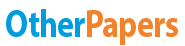# Financial Ratios

Essay by   •  August 6, 2011  •  Essay  •  358 Words (2 Pages)  •  1,232 Views

## Essay Preview: Financial Ratios

Report this essay
Page 1 of 2

Financial Ratios

Financial ratios are useful indicators of a firm's performance and financial situation. Most ratios can be calculated from information provided by the financial statements. Financial ratios can be used to analyze trends and to compare the firm's financials to those of other firms. In some cases, ratio analysis can predict future bankruptcy.

Financial ratios can be classified according to the information they provide. The following types of ratios frequently are used:

* Liquidity ratios

* Asset turnover ratios

* Financial leverage ratios

* Profitability ratios

* Dividend policy ratios

Liquidity Ratios

Liquidity ratios provide information about a firm's ability to meet its short-term financial obligations. They are of particular interest to those extending short-term credit to the firm. Two frequently-used liquidity ratios are the current ratio (or working capital ratio) and the quick ratio.

The current ratio is the ratio of current assets to current liabilities:

Current Ratio = Current Assets

Current Liabilities

Short-term creditors prefer a high current ratio since it reduces their risk. Shareholders may prefer a lower current ratio so that more of the firm's assets are working to grow the business. Typical values for the current ratio vary by firm and industry. For example, firms in cyclical industries may maintain a higher current ratio in order to remain solvent during downturns.

One drawback of the current ratio is that inventory may include many items that are difficult to liquidate quickly and that have uncertain liquidation values. The quick ratio is an alternative measure of liquidity that does not include inventory in the current assets. The quick ratio is defined as follows:

Quick Ratio = Current Assets - Inventory

Current Liabilities

The current assets used in the quick ratio are cash, accounts receivable, and notes receivable. These assets essentially are current assets less inventory. The quick ratio often is referred to as the acid test.

Finally, the cash ratio is the most conservative liquidity ratio. It excludes all current assets except the most liquid: cash and cash equivalents. The cash ratio is defined as follows:

Cash Ratio = Cash + Marketable Securities

...

...